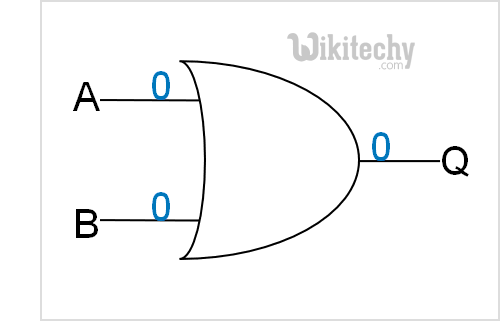# apache hive - Hive Built in Operators - hive tutorial - hadoop hive - hadoop hive - hiveql

## What is an Operators in Hive?

• An operator is an object that is capable of manipulating a value or operator.
• For example, in "1 + 2", the "1" and "2" are the operands and the plus symbol is the operator.
• Booleans are also considered operators where AND, OR, and NOT can also be used in most programming languages.

## There are four types of operators in Hive:

• Arithmetic Operators
• Relational Operators
• Logical Operators
• Complex Operators

## Arithmetic Operators in Hive

• These operators support various common arithmetic operations on the operands.
• All of them return number types.
Operator Operand Description
A = B all primitive types TRUE if expression A is equivalent to expression B otherwise FALSE.
A != B all primitive types TRUE if expression A is not equivalent to expression B otherwise FALSE.
A < B all primitive types TRUE if expression A is less than expression B otherwise FALSE.
A <= B all primitive types TRUE if expression A is less than or equal to expression B otherwise FALSE.
A > B all primitive types TRUE if expression A is greater than expression B otherwise FALSE.
A >= B all primitive types TRUE if expression A is greater than or equal to expression B otherwise FALSE.
A IS NULL all types TRUE if expression A evaluates to NULL otherwise FALSE.
A IS NOT NULL all types FALSE if expression A evaluates to NULL otherwise TRUE.
A LIKE B Strings TRUE if string pattern A matches to B otherwise FALSE.
A RLIKE B Strings NULL if A or B is NULL, TRUE if any substring of A matches the Java regular expression B , otherwise FALSE.
A REGEXP B Strings Same as RLIKE.

## Example

• The following query adds two numbers, 25 and 35.

## Result:apache hive - learn hive - hive tutorial - arithmetic operator - hive example

## Relational Operators in Hive:

• These operators are used to compare two operands.

## To give below table describes the Relational operators available in Hive:

Operators Operand Description
A + B all number types Gives the result of adding A and B.
A - B all number types Gives the result of subtracting B from A.
A * B all number types Gives the result of multiplying A and B.
A / B all number types Gives the result of dividing B from A.
A % B all number types Gives the reminder resulting from dividing A by B.
A & B all number types Gives the result of bitwise AND of A and B.
A | B all number types Gives the result of bitwise OR of A and B.
A ^ B all number types Gives the result of bitwise XOR of A and B.
~A all number types Gives the result of bitwise NOT of A.

## Example

• Assume the wikitechy_employee table is composed of fields named Id, Name, Salary, Designation, and Dept.
• Generate a query to retrieve the wikitechy_employee details whose Id is 2205.

## Logical Operators

• The operators are logical expressions.All of them return either TRUE or FALSE.
Operators Operands Description
A AND B boolean TRUE if both A and B are TRUE, otherwise FALSE.
A && B boolean Same as A AND B.
A OR B boolean TRUE if either A or B or both are TRUE, otherwise FALSE.
A || B boolean Same as A OR B.
NOT A boolean TRUE if A is FALSE, otherwise FALSE.
!A boolean Same as NOT A.

## Example

• Below query is used to retrieve employee details whose Department is TP and Salary is more than Rs 40000.

## Outputapache hive - learn hive - hive tutorial - logical operator - hive example

## Complex Operators

• These operators provide an expression to access the elements of Complex Types.
Operator Operand Description
A[n] A is an Array and n is an int It returns the nth element in the array A. The first element has index 0.
M[key] M is a Map<K, V> and key has type K It returns the value corresponding to the key in the map.
S.x S is a struct It returns the x field of S.